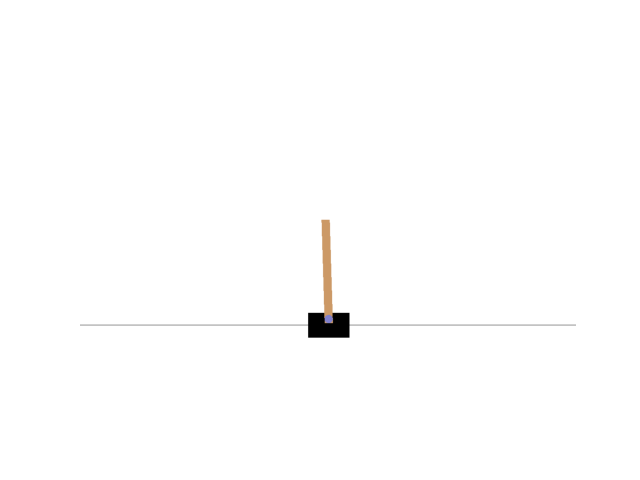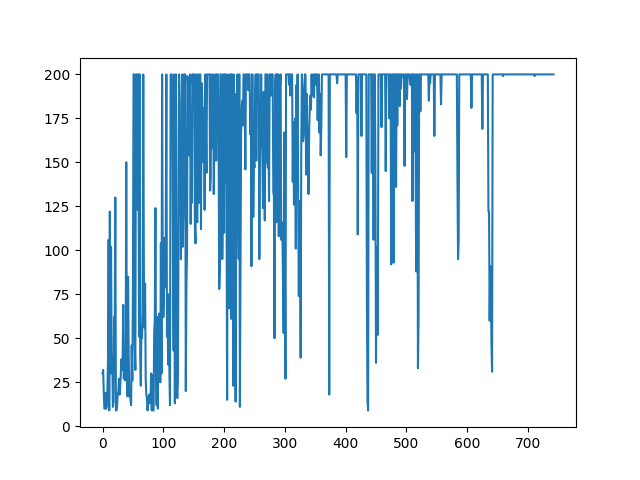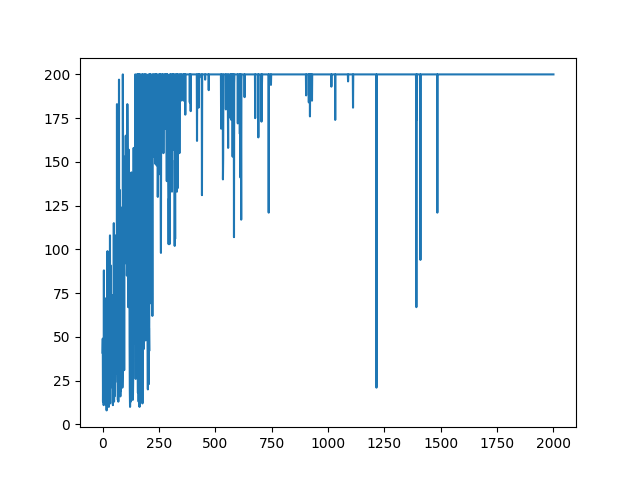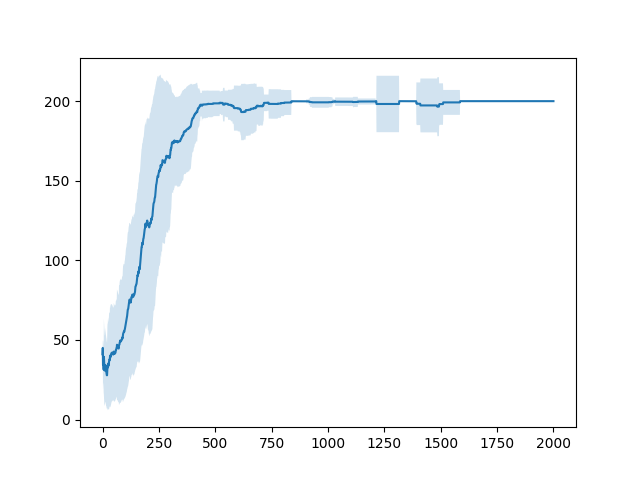# Introduction

I wrote Article about Q-learning before. As a concrete example, I introduced the two-bowl bandit problem, but since it does not evolve in time (the state does not change), I will introduce the inverted pendulum problem, which is a problem with time evolution. You don't need to read it as it is independent of the previous article. The code was written in Python 3.8.6.

# ε-greedy method

First, the ε-greedy method will be explained. Since the Q value is randomly generated in the initial stage of learning, it may not be possible to learn well because only fixed actions that depend on the initial value are performed (a large value with a Q value is generated, and the fixed action at that point. Not etc.). The ε-greedy method is one of the methods for solving the above problems. Specifically, a random action is selected with a probability ε, and an action with the maximum Q value is selected with a probability \$ (1- \ epsilon) \$. There is a trade-off between taking a random action and taking an action that maximizes the Q value, and as the number of random actions increases, learning does not proceed well and the Q value becomes maximum. If the number of such actions increases, a wide range of searches will not be possible. Ideally, in the first half of learning, many random actions should be taken to search for a lot, and in the end of learning, many actions should be taken to maximize the Q value to increase utilization. Therefore, it is possible to learn more efficiently by reducing the value of ε as the learning progresses.

CartPole CartPole is one of the game environments provided by OpenAI Gym and is a game related to Inverted Pendulum. The inverted pendulum problem is a problem in which a rod having a fixed rotation axis is erected on a trolley and the trolley is moved left and right to control the rod so that it does not fall. The state of Cart Pole is as follows.Install OpenAI Gym as follows.

``````pip install gym
``````

After installation, run the following code to see if it works.

``````import gym
#Environment generation
env = gym.make('CartPole-v0')
#Early in the environment
observation = env.reset()
for t in range(1000):
#To display the current status
env.render()
#The return value to make the sample act is the state of the dolly and stick, the reward obtained, the game end flag, detailed information from the left
observation, reward, done, info = env.step(env.action_space.sample())
if done:
print("Finished after {} timesteps".format(t+1))
break
#Close environment
env.close()
``````

In my case (Mac OS Big Sur ver11.1) ImportEroor occurred, but I could solve it by installing the following library.

``````pip install pyglet==1.5.11
``````

Next, CartPole in OpenAI Gym will be explained. First, the conditions for the dolly and rod are as follows. __ ・ Bogie position: \$ -2.4 \ sim2.4 \$ ・ Bogie speed: \$-\ inf \ sim \ inf \$ ・ Bar angle: \$ -41.8 \ sim 41.8 \$ ・ Velocity of the tip of the rod: \$-\ inf \ sim \ inf \$ __ All of these initial values ​​are randomly generated numbers between \$ -0.05 \ sim 0.05 \$. There are two actions for this environment. __1. Push the dolly to the left 2. Push the dolly to the right __ The reward is given "1" for each step, and the conditions for ending the episode are the following three. __1. The absolute value of the bar angle is 12 ° or more 2. The absolute value of the position of the dolly is 2.4 or more 3. The episode exceeds 200 steps. __ The condition for considering learning as complete is when a reward of 195 or more is obtained in 100 consecutive trials. If you want to know more accurately, look at the condition source this.

## Implementation (Q-learning)

Create a Q table (a table in which Q values ​​corresponding to each state and each action are written) so that the stick does not fall during Q learning. Implementation is divided into main, agent, environment, and state (brain).

### State (brain)

First, the code related to the state will be explained. Here, the Q table is updated, actions are selected, and the observed state is discretized. Discretization of the observed state is performed because if the information is as it is, it has continuous values ​​and there are innumerable states, which increases the size of the Q table and makes learning difficult.

#### `state.py`

``````
import numpy as np

#Number of divisions in each state
NUM_DIZITIZED = 6

#Learning parameters
GAMMA = 0.99  #Time discount rate
ETA = 0.5  #Learning coefficient

class State:
def __init__(self, num_states, num_actions):
#Get the number of actions
self.num_actions = num_actions

#Create Q table(Division number^Number of states)×(Number of actions)
self.q_table = np.random.uniform(low=-1, high=1, size=(
NUM_DIZITIZED**num_states, num_actions))

def bins(self, clip_min, clip_max, num):
#Find the threshold for digital conversion of the observed state
return np.linspace(clip_min, clip_max, num + 1)[1:-1]

def analog2digitize(self, observation):
#Discretization of states
cart_pos, cart_v, pole_angle, pole_v = observation
digitized = [
np.digitize(cart_pos, bins=self.bins(-2.4, 2.4, NUM_DIZITIZED)),
np.digitize(cart_v, bins=self.bins(-3.0, 3.0, NUM_DIZITIZED)),
np.digitize(pole_angle, bins=self.bins(-0.5, 0.5, NUM_DIZITIZED)),
np.digitize(pole_v, bins=self.bins(-2.0, 2.0, NUM_DIZITIZED))
]
return sum([x * (NUM_DIZITIZED**i) for i, x in enumerate(digitized)])

def update_Q_table(self, observation, action, reward, observation_next):
#Discretization of states
state = self.analog2digitize(observation)
state_next = self.analog2digitize(observation_next)
Max_Q_next = max(self.q_table[state_next][:])
#Update Q table(Q learning)
self.q_table[state, action] = self.q_table[state, action] + \
ETA * (reward + GAMMA * Max_Q_next - self.q_table[state, action])

def decide_action(self, observation, episode):
# ε-Select an action by the greedy method
state = self.analog2digitize(observation)
epsilon = 0.5 * (1 / (episode + 1))

if epsilon <= np.random.uniform(0, 1):
#Take the most valuable action.
action = np.argmax(self.q_table[state][:])
else:
#Act appropriately.
action = np.random.choice(self.num_actions)
return action
``````

The initial value of the Q table is randomly generated from -1 to 1 (0 to 1 is also acceptable). The processing related to discretization is performed according to the conditions of the carriage and the rod. Adopt the ε-greedy method introduced earlier so that ε becomes smaller as learning progresses.

### Agent

In the code related to the agent, the code in the previous state is called to update the Q table and select the action.

#### `agent.py`

``````
from state import State

class Agent:
def __init__(self, num_states, num_actions):
#Generate environment
self.state = State(num_states, num_actions)

def update_Q_function(self, observation, action, reward, observation_next):
#Update Q table
self.state.update_Q_table(observation, action, reward, observation_next)

def get_action(self, observation, step):
#Action
action = self.state.decide_action(observation, step)
return action
``````

### environment

In the code related to the environment, learning is performed by calling the code of the agent by adding new processing related to drawing for each episode, such as the condition of learning completion, the play video of the model finally created, and the reward. The number of trials was set to 1000, but if learning is not completed, it is better to increase the number of trials.

#### `env.py`

``````
import numpy as np
import matplotlib.pyplot as plt
from matplotlib import animation

import gym

from agent import Agent

#Maximum number of steps
MAX_STEPS = 200
#Maximum number of attempts
NUM_EPISODES = 1000

class Environment():
def __init__(self, toy_env):
#Generate environment
self.env = gym.make(toy_env)
#Get the number of states
num_states = self.env.observation_space.shape
#Get the number of actions
num_actions = self.env.action_space.n
#Generate Agent
self.agent = Agent(num_states, num_actions)

def run(self):
complete_episodes = 0 #Number of successes
step_list = []
is_episode_final = False  #Last attempt
frames = []  #Variable to save the image

#Repeat for a few trials
for episode in range(NUM_EPISODES):
observation = self.env.reset()  #Environment initialization
for step in range(MAX_STEPS):
#Save the image only for the last attempt.
if is_episode_final:
frames.append(self.env.render(mode='rgb_array'))

#Seeking action
action = self.agent.get_action(observation, episode)
#Action a_By executing t, s_{t+1}, r_{t+1}Seeking
observation_next, _, done, _ = self.env.step(action)

#Give a reward
if done:  #Done becomes true when 200 steps have passed or when tilted more than a certain angle
if step < 195:
reward = -1  #Because I failed-Give 1 reward
complete_episodes = 0  #Reset the number of successes
else:
reward = 1  #Because it was successful+Give 1 reward
complete_episodes += 1  #Break continuous success record
else:
reward = 0
#Update Q table
self.agent.update_Q_function(observation, action, reward, observation_next)
#Observation update
observation = observation_next

#Processing at the end
if done:
step_list.append(step+1)
break

if is_episode_final:
es = np.arange(0, len(step_list))
plt.plot(es, step_list)
plt.savefig("cartpole.png ")
plt.figure()
patch = plt.imshow(frames)
plt.axis('off')

def animate(i):
patch.set_data(frames[i])

anim = animation.FuncAnimation(plt.gcf(), animate, frames=len(frames),
interval=50)
anim.save('movie_cartpole_v0.mp4', "ffmpeg")
break

#Make the final attempt after 10 consecutive successes
if complete_episodes >= 100:
print('100 consecutive successes')
is_episode_final = True
``````

### Main

Call the environment code to learn.

#### `main.py`

``````
from env import Environment

TOY = "CartPole-v0"

def main():
cartpole = Environment(TOY)
cartpole.run()

if __name__ == "__main__":
main()
``````

### result

Finally, I was able to control the CartPole as follows.The rewards changed as follows.In this code, learning is stopped when learning is completed, but if you continue without stopping, the reward will be as follows.It turns out that it sometimes fails over time. Next, let's look at the average reward and its variability (standard deviation) for the last 100 episodes.It turns out that this is more intuitive to judge whether or not learning is possible.